# Number Sequences ExplanationNumber series - common patterns

On this page we will discuss the most common patterns and explain how you can recognize these patterns in the exercises. Read this page carefully before you start practicing, this will make practicing much more effective! In the assessment you will eventually do, there may be even more patterns than are covered here, but after reading this page you are prepared for the most common patterns.

If we apply a helicopter view to the number series, there are roughly six different types of main patterns. Within these patterns there are sometimes many different possibilities, but it is important to see as early as possible what kind of main pattern is most likely to apply to an exercise. The following patterns are common:

1. Monotone Pattern - The differences between consecutive numbers are very similar in size, and the same action is always taken to arrive at the next number. It is almost always about adding or subtracting.
2. Exponential pattern - The differences between consecutive numbers get bigger or smaller quickly, but the same action is always done to get to the next number. It is almost always about multiplication or division.
3. Alternating Pattern - The above patterns are alternated. For example, from number 1 to number 2 in the series there is addition, but from number 2 to number 3 the number is multiplied to find the next number in the series.
4. Two Sequences in One - In this pattern, there are actually two sequences that are intertwined. For example, number 1,3 and 5 are a series and number 2,4 and 6 are a series.
5. Exceptions - For example, a Fibonacci sequence, where a number is built from the two previous numbers in the sequence.
6. Fractions - Often two strings are applied here, one above the division line and one below the division line. These patterns can be very complicated.

Of course there are also series that fall a bit between the different main dressings, but these are also covered on this page to make sure you will recognize them more and more.

Monotone relation

In a monotone pattern, the steps between the different numbers are approximately or exactly the same size. In addition, the numbers will either get bigger or smaller. Since the steps are always about the same size, there will only be addition or subtraction. A simple example of a monotone connection is:

1 3 5 7 ?

Not many people will have trouble finding the pattern in the example above, the answer is 9. Also a monotone deduction is relatively easy to spot.

15 12 9 6 ?

The answer is 3. However, variations on a monotone relationship are possible, sometimes making it more difficult to recognize. In some cases, a monotone series almost resembles an exponential relationship. For example, look at the next series.

2 6 15 29 ?

You can see here that the numbers are getting bigger faster and faster and you might think that there is an exponential pattern. However, this is not the case. You can see this because the numbers are clearly not multiplied by a constant other number. Therefore, draw the addition arcs to see the pattern more clearly.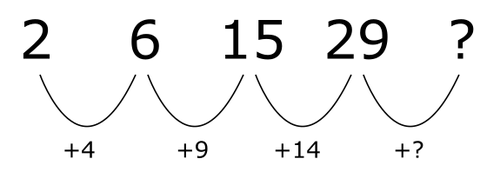You already see a series emerging where the difference is increasing with 5 every step, so the answer is 48.

You can also have a series where the difference is getting smaller. For example:

2 17 30 41 ?

You solve this series in the same way.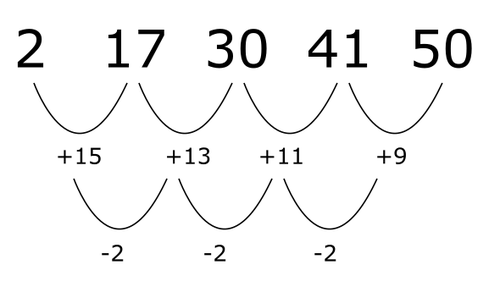You can also encounter the same type of sequence with a subtraction pattern. For example in the following pattern.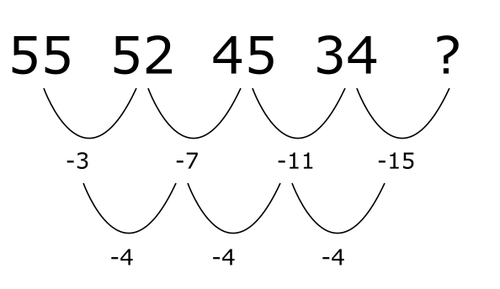The answer is 34 - 15 = 19.

Exponential relation

In an exponential pattern, the numbers will get bigger or smaller, but the differences between two consecutive numbers in the series will also get bigger every step (when multiplying) or less (when dividing by something) ). The simplest example of an exponential relationship is:

2 4 8 16 ?

Most people will see that every step the previous number is multiplied by 2, so the answer is 32. The same is true if we reverse the sequence:

32 16 8 4 ?

It is now clear that the previous number is always divided by 2. However, there are also variations on the pattern, making it more difficult to spot.

1 2 8 48 ?

You can see that there is probably an exponential pattern. The differences keep getting bigger and the step from 1 to 2 is of a completely different order than from 8 to 48. You now write down the multiplication arcs. Be precise in this, because you make mistakes easily. You will now see the pattern emerge.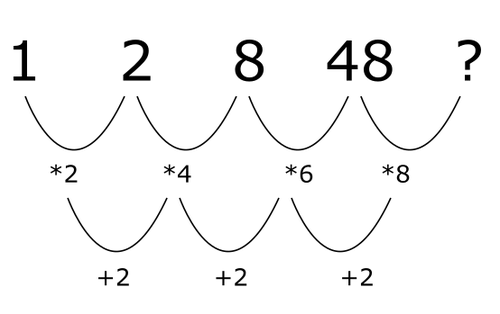The answer is 384. There are other variations of the exponential pattern:

3 14 58 234 ?

You again see that there must be an exponential pattern. However, you also immediately see that you cannot divide 14 by 3. Writing the multiplication arcs will not help you now. This is because to find the next number in a series, the previous number is multiplied by a number, but in addition, a number is always added. In this case, the sequence is of the following form: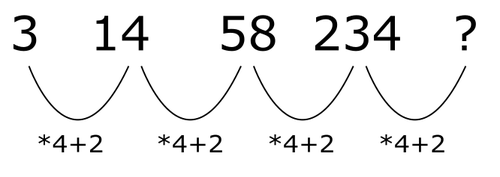However, this is quite difficult to see for most people. If you don't see the pattern right away, there is a way to make this easier for yourself. First write the addition arcs and then write the multiplication arcs. Because the same number is always added, you can see with which number it is multiplied.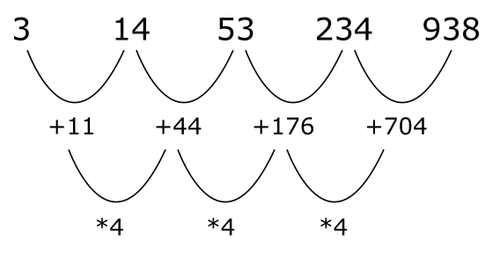Of course, these types of patterns are also possible with a divided by pattern. See for example:

440 216 104 48 ?

This pattern has the following basic shape.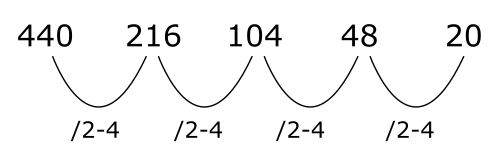You can find this pattern by drawing the following arcs: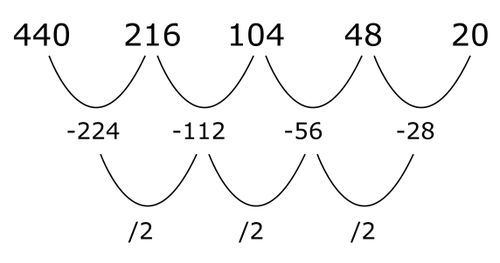It gets even more complicated when you alternate patterns. Actually, these types of patterns belong in the following topic, but we will discuss one here:

2 7 10 23 42 ?

You can see that this pattern probably involves multiplication, although it is not immediately obvious. If you write addition arrows, you don't get much wiser. If you look a bit through your eyelashes, you can see that there is approximately multiplied by two. This allows you to recognize the following pattern: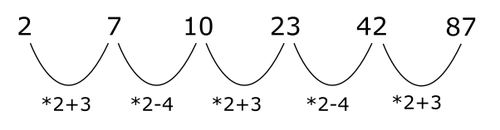This is a difficult pattern, but it can certainly occur in your assessment!

Alternating pattern

In an alternating pattern, the above patterns are alternated. Sometimes even three different patterns are alternated. With these types of patterns you will often have no idea at first what happens to the numbers. It is then important to write down the curves neatly. As a number increases, you can see if it multiplies or adds in that step. If a number decreases by one step, you can see whether there is a subtraction or divided by.

For example:

4 2 6 4 12 ?

from the 1st number in the series to the 2nd the number increases, the transformation could be -2 or / 2. From 2nd number to the 3rd it could be * 3 or +4. From the 3rd number to the 4th the only possibility is -2 From the 3rd to 4th number the possible transformations are +8 or * 3. It looks like the pattern is found that way!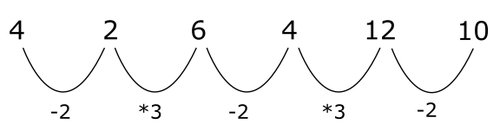An example where three patterns are alternated:

9 27 36 12 ?

You can see that it is likely that * 3 then +9 and then / 3 are applied first. The logical answer is now 36. There could also have been +18 in the first step, then the answer would be 40. It is therefore important to look carefully at the possible answers.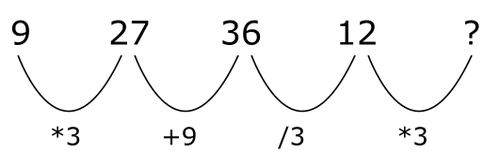Or is the following pattern applied?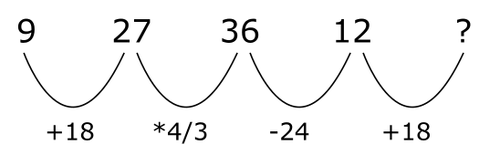If you have a short sequence with an alternating pattern, sometimes you are not sure which action is being performed, as the example above shows. Therefore, look carefully at the possible answers and always try the most logical option first. For example, above it makes no sense that from 2nd to the 3rd number in the series the number is multiplied by 4/3, it makes more sense to consider +9 first.

There are many more different possibilities of alternating patterns, but they are all based on the same principle. It is good to practice a lot. With Assessly you can practice as much as you want, for free.

Two series in one

With this type of pattern, there are actually two patterns that intertwine. If you go through the numbers chronologically, you will start to panic a bit. This is because the strings are independent of each other. An example:

8 16 32 8 128 4 ?

If you look at the first 3 numbers, you think you have found an easy pattern. However, this will put you on the wrong track. The arcs look like this: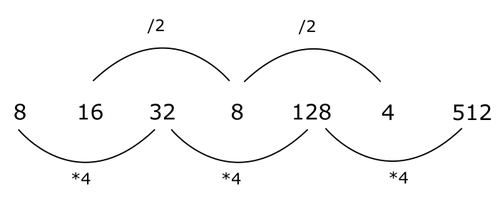Many different variations of this pattern are possible. We have a lot of practice assignments that contain these kinds of patterns, so you can all practice them!

Exceptions

To put you on the wrong track, there are often a few “exception patterns” in an assessment. We've worked out some common exceptions below to make it easier to spot them in your assessment.

Fibonacci

In a fibonacci sequence, the numbers are built up by adding the two previous numbers. This series is relatively easy to recognize and is also quite common. So it is one that you should always look out for.

1 2 3 5 8 13 21 etc

Or the variant in which the three previous numbers are added:

0 1 2 3 6 11 20 etc

Subtract previous number

Here the third number is found by subtracting the second number from the first number. Actually, this is a kind of reverse fibonacci. In this sequence, the numbers sometimes jump in all directions (especially if it contains a negative number), so don’t panic, but check for this pattern. For example:

12 16 4 -12 -16 -4 12 etc

Divide by previous number

Here the next number in the series is found by dividing the two previous numbers. For example:

3 6 2 ⅓ ⅙ ½ 3 etc

Multiply by the previous number

Same principle, for example:

1 2 2 4 8 32 256 etc

Exponential

A bit confusing because we've talked about exponential series before. In this case it concerns, for example, the following series:

4 16 36 64 100 144 196 etc

You can see that this concerns the following series:

22 42 62 82 102 122 142 etc

You can scan for the common squares and if you recognize them you know almost immediately that you are dealing with such a series. What you can also have is a small edit on this string. For example, think of something like:

2 14 34 62 98 142 194 etc

This way you don't immediately recognize the squares, but you can see that the sequence has the following pattern.

22-2 42-2 62-2 82-2 102-2 122-2 142-2 etc

In addition, for this type of pattern there is an easy trick to calculate the next number. Write the addition arcs twice and you have found the pattern without squaring numbers.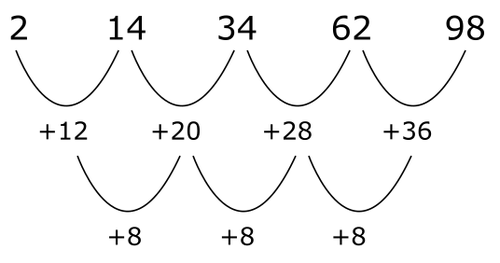Very nasty exceptions

Sometimes there is a question in the series to test whether you can think out of the box. You should especially look for these patterns if you cannot recognize any of the other patterns. It is important to take a good look at the possible multiple choice answers. Consider, for example, the following patterns:

Numbers are getting bigger / smaller

Example:

2 3 8 9 13 48 ?

With the multiple choice options you will now only see 1 number that is greater than 48. So don't panic because you don't find a pattern and just choose that number.

Even / odd numbers only

Example:

8 4 32 -1284 36 ?

The same applies to this. If you are pretty sure that no other pattern applies, you should take a good look at the multiple choice answers.

Patterns with the number of letters in a word

Example:

Bus Dog Hats Strength

You can see the number of letters in the word always increases by one, so you should select the answer that meets that criterium.

Fractions

Many people struggle with patterns with fractions. It is important to know the calculation rules for fractions as you learned them in elementary school a long time ago. Furthermore, the series with fractions can be roughly divided into two categories.

1. Normal patterns with fractions
2. A pattern above the division line and a pattern below the division line.

“Normal patterns” that contain fractures

In this type of pattern, one of the previously mentioned patterns is applied, but then to fractions. For example:

⅛ ¼ ½ ?

It is clear that there is always multiplied by 2, the answer is 1.

Or for example

½ ¾ ⅝ ⅞ ?

By neatly writing the addition arcs, you will see the following sequence arise: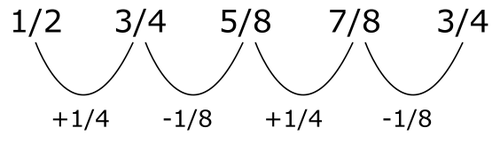So do not panic immediately when you see fractions, often it will just be a normal pattern. It is important to see at the earliest possible stage whether you should look for a normal pattern, or if you are looking for a pattern above the division line and a pattern below the division line.

A pattern above the division line and a pattern below the division line.

Difficult patterns can be involved here. Always scan a fraction series for "illogical" fractions. Sometimes there will be 3/12 for example. Then you immediately have to wonder why there is no ¼. The number might be put there to trick you, so it's good to spot that early on. It is sometimes important to write the fractions in your head in different ways, otherwise it is sometimes impossible to solve a sequence with a pattern above and below the division line.

For example: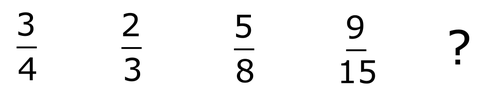The first thing to notice is 9/15. Why is there no ⅗ here? Furthermore, at first sight there is no clear pattern, so for all other fractions we also have to look at how best to write them.

The first number shows that we divide by 4 and the third number we see that we divide by 8. Can we rewrite the second and fourth numbers to fit this pattern? In the following way it is actually the same and suddenly we see clearly what the patterns are: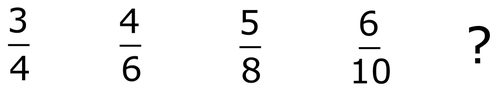Above the line 1 is always added and below the line 2 is always added.

These types of series can become a lot more complicated, but you will not encounter many in most assessments. A brain teaser: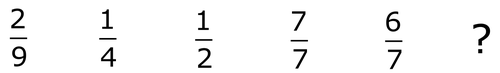It is now a question of how to rewrite the fractions. You will immediately see that 7/7 is equal to 1. So you can write it as you want if above the line but the same number as below the line. ¼ can be rewritten as 2/8 or 3/12 or 4/16. ½ can also be written as 2/4, 3/6, 4/8 etc. If you rewrite the sequence like this, you will see the pattern emerge.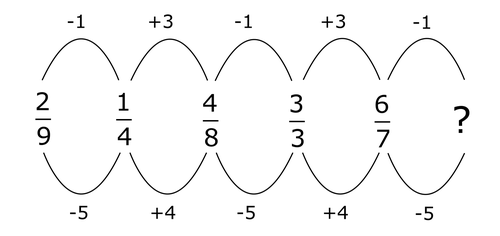So the answer is 5/2 or 2 ½. It is often a bit of a search with this type of series. Do not linger too long, because then you will have too little time for the other exercises in the assessment.

Good luck with practicing and of course with the final assessment!

## Number Sequences practice sets